Python基础语法入门篇(二)

# 1. 注释

## 1.1 单行注释

``````# 输出hello world
print("hello world")# 输出hello world
``````

`Shift + 3`，或者`Ctrl + ? `都可以生成#号

## 1.2 多行注释

``````
'''
^^^^^^^^^^^^^^^^^^^^^^^^^^^^^^^^^^^^^^^^^^^^^
佛祖保佑 永无BUG
佛曰:
写字楼里写字间，写字间里程序员；
程序人员写程序，又拿程序换酒钱。
酒醒只在网上坐，酒醉还来网下眠；
酒醉酒醒日复日，网上网下年复年。
但愿老死电脑间，不愿鞠躬老板前；
奔驰宝马贵者趣，公交自行程序员。
别人笑我忒疯癫，我笑自己命太贱；
不见满街漂亮妹，哪个归得程序员？
'''
``````

# 2.变量以及数据类型

## 2.1 变量的定义

``````# 定义一个变量表示这个字符串。如果需要修改内容，只需要修改变量对应的值即可
# 注意，变量名不需要使用引号包裹
weather = "今天天气真好"
print(weather)
print(weather)
print(weather)
``````

1）变量即是可以变化的量，可以随时进行修改。

2）程序就是用来处理数据的，而变量就是用来存储数据的。

## 2.2 变量的类型

Python 里为了应对不同的业务需求，也把数据分为不同的类型。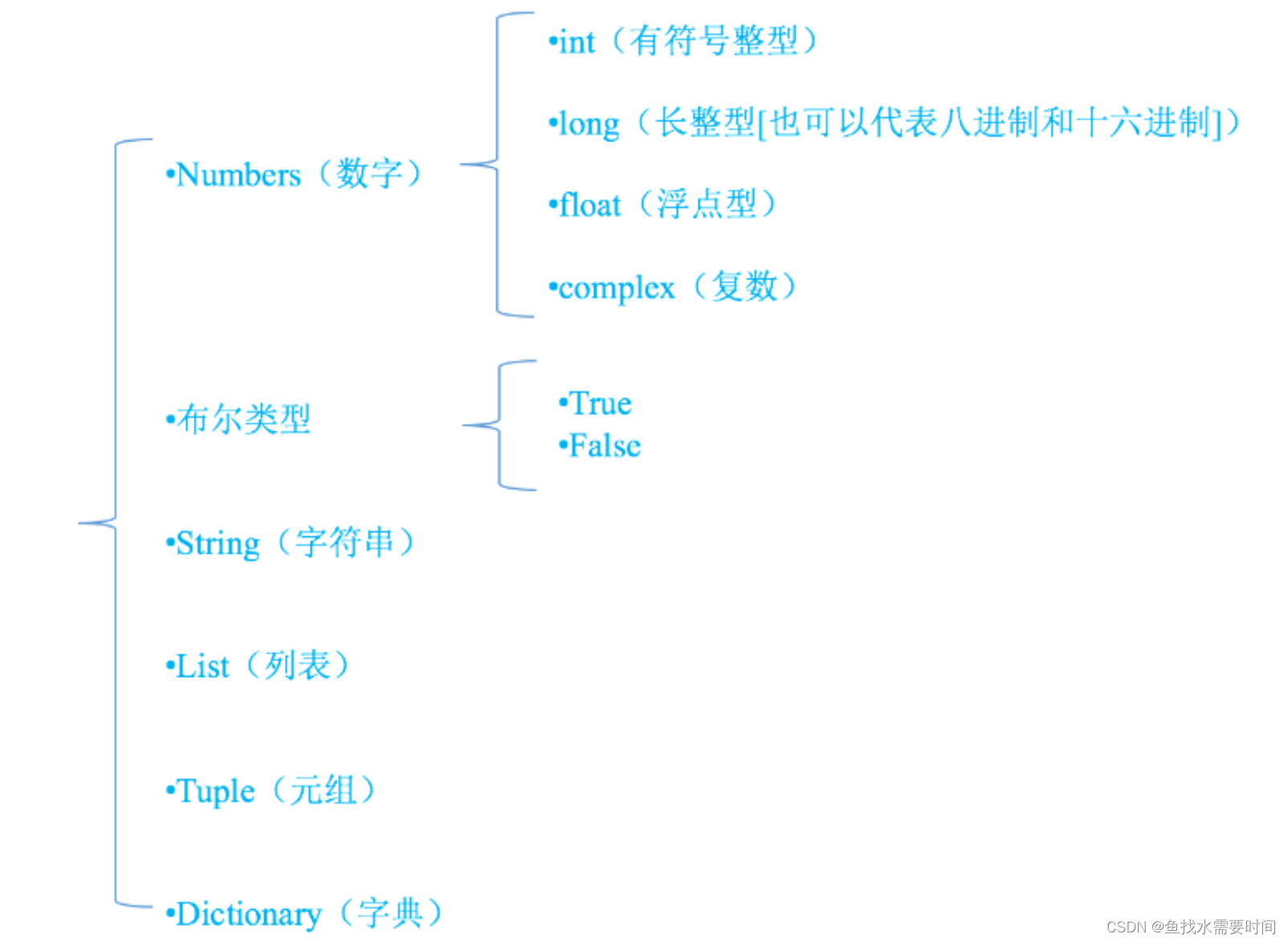python中没有double类型，也没有char 字符类型

Tuple：和List类似，用一个数据代表很多数据的集合

Dictionary：和现实中字典功能类似

``````# int
money = 100
print(100)
# float
price = 99.9
print(price)
# boolean
# 变量要见名识意，不要用中文拼音，会被人看不起
# 男 True，女 False
sex = True
print(sex)
# String
# 字符串使用单引号或双引号都可以，但是必须成对出现
str = 'hello '
str2 = 'world'
print(str + str2)
# 单引号和双引号的嵌套
str3 = '"哈哈"'
str4 = "'哈哈'"
print(str3)
print(str4)
# 单引号和双引号会就近匹配，相同的不可以嵌套
# str5 = ""嘿嘿""  错误用法
``````
``````# list
name_list = ['张飞', '关羽']
print(name_list)
# 获取list中下标为0的字符串，下标从0开始计算：0、1、2、3....
print(name_list)
# tuple
age_tuple = (18, 19, 20)
print(age_tuple)
# dictionary 字典
person = {'name': '张三', 'age': 18}
print(person)
``````

## 2.3 查看数据类型

``````str5 = "hello"
print(type(str5))
#<class 'str'>
#str是string的一个缩写
``````

# 3.标识符和关键字

1. 标识符由字母、下划线和数字组成(\$等符号都不可以)，且不能以数字开头

2. 严格区分大小写。

3. 不能使用关键字

## 3.1 命名规范

• 标识符命名要做到顾名思义。
• 起一个有意义的名字，尽量做到看一眼就知道是什么意思(提高代码可 读性) 比如: 名字 就定义为 name , 定义学生用 student

``````# 正确示例
name = "张三"
age = 18
#错误命名
a = "张三"
b = 18
``````
• 遵守一定的命名规范。
• 驼峰命名法，又分为大驼峰命名法和小驼峰命名法。
•2）大驼峰式命名法（upper camel case）： 每一个单字的首字母都采用大写字母，例如：FirstName、LastName.

3）还有一种命名法是用下划线“_”来连接所有的单词，比如send_buf.

​ Python的命令规则遵循PEP8标准

## 3.2 关键字

• 关键字的概念
• 一些具有特殊功能的标识符，这就是所谓的关键字。

关键字，已经被python官方使用了，所以不允许开发者自己定义和关键字相同名字的标识符。

• 关键字
• ``````False None True and as assert break class continue def del elif else except
finally for from global if import in is lambda nonlocal not or pass raise
return try while with yield
``````

# 4. 类型转换

函数 说明 说明
int(x) 将x转换为一个整数
float(x) 将x转换为一个浮点数
str(x) 将对象 x 转换为字符串
bool(x) 将对象x转换成为布尔值

转换成为整数

``````print(int("123")) # 123 将字符串转换成为整数
print(int(123.78)) # 123 将浮点数转换成为整数

print(int(True)) # 1 布尔值True转换成为整数是 1
print(int(False)) # 0 布尔值False转换成为整数是 0

# 以下两种情况将会转换失败
'''
123.456 和 12ab 字符串，都包含非法字符，不能被转换成为整数，会报错
print(int("123.456"))
print(int("12ab"))
'''
``````

转换成为浮点数

``````f1 = float("12.34")
print(f1) # 12.34
print(type(f1)) # float 将字符串的 "12.34" 转换成为浮点数 12.34

f2 = float(23)
print(f2) # 23.0
print(type(f2)) # float 将整数转换成为了浮点数
``````

转换成为字符串

``````str1 = str(45)
str2 = str(34.56)
str3 = str(True)  #True
print(type(str1),type(str2),type(str3))
``````

转换成为布尔值

``````print(bool(1)) #True，非0的整数都是True
print(bool(' ')) #True，空格也是True
print(bool(0.1)) #True，非0的浮点数都是True

# 以下情况都是False
print(bool(0)) #False
print(bool(0.0)) #False
print(bool('')) #False，没有内容的字符串都是False
print(bool("")) #False

print(bool({})) #False，只要字典中有数据，强制转换为bool，就返回True
print(bool([])） #False，只要列表中有数据，强制转换为bool，就返回True
print(bool(())） #False只要元组中有数据，强制转换为bool，就返回True

tuple1 = (0)
print(bool(tuple1)) #False
tuple2 = (0, 0)
print(bool(tuple)) #True
``````

# 5. 运算符

## 5.1 算数运算符

以a=10 ,b=20为例进行计算

运算符 描述 实例
+ 两个对象相加 a + b 输出结果 30
得到负数或是一个数减去另一个数 a – b 输出结果 -10
* 两个数相乘或是返回一个被重复若干次的字符串 a * b 输出结果 200
/ b / a 输出结果 2
// 取整除 返回商的整数部分 9//2 输出结果 4 , 9.0//2.0 输出结果 4.0
% 取余 返回除法的余数 b % a 输出结果 0
** 指数(幂) a**b 为10的20次方
() 小括号 提高运算优先级，比如: (1+2) * 3

注意：混合运算时，优先级顺序为： ** 高于 * / % // 高于 + – ，为了避免歧义，建议使用 () 来处理运

算符优先级。 并且，不同类型的数字在进行混合运算时，整数将会转换成浮点数进行运算。

``````>>> 10 + 5.5 * 2
21.0
>>> (10 + 5.5) * 2
31.0
``````

## 5.2 算数运算符在字符串里的使用

注意：在python中， + 两端都是字符串才可以进行拼接字符串，不是字符串类型的数据可以通过str()强转为字符串 ，再进行拼接

字符串的乘法，是将字符串重复多少次

``````print('11' + '22')  #1122
print(' hello world' * 3) # hello world hello world hello world
``````

## 5.3 赋值运算符

运算符 描述 实例
= 赋值运算符 把 = 号右边的结果 赋给 左边的变量，如 num = 1 + 2 * 3，结果num的值为7
``````# 同时为多个变量赋值(使用等号连接)
>>> a = b = 4
>>> a
4
>>> b
4

>>> # 多个变量赋值(使用逗号分隔)
>>> num1, f1, str1 = 100, 3.14, "hello"
>>> num1
100
>>> f1
3.14
>>> str1
"hello"
``````

## 5.4 复合赋值运算符

运算符 **描述 **实例
+= 加法赋值运算符 c += a 等效于 c = c + a
-= 减法赋值运算符 c -= a 等效于 c = c – a
*= 乘法赋值运算符 c *= a 等效于 c = c * a
/= 除法赋值运算符 c /= a 等效于 c = c / a
//= 取整除赋值运算符 c //= a 等效于 c = c // a
%= 取模赋值运算符 (取余，模余) c %= a 等效于 c = c % a
**= 幂赋值运算符 c **= a 等效于 c = c ** a
``````# 示例：+=
>>> a = 100
>>> a += 1 # 相当于执行 a = a + 1
>>> a
101

# 示例：*=
>>> a = 100
>>> a *= 2 # 相当于执行 a = a * 2
>>> a
200

# 示例：*=，运算时，符号右侧的表达式先计算出结果，再与左边变量的值运算
>>> a = 100
>>> a *= 1 + 2 # 相当于执行 a = a * (1+2)
>>> a
300
``````

## 5.5 比较运算符

以下假设变量a为10，变量b为20：

运算符 描述 示例
== 等于:比较对象是否相等(也叫恒等) (a == b) 返回 False
!= 不等于:比较两个对象是否不相等(python2中 <> 代表不等于) (a != b) 返回True
> 大于:返回x是否大于y (a > b) 返回False
>= 大于等于:返回x是否大于等于y (a >= b) 返回False
< 小于:返回x是否小于y。所有比较运算符返回1表示真，返回0表示假。这分别与特殊的变量True与False等价 (a < b) 返回True
<= 小于等于:返回x是否小于等于y (a <= b) 返回True

## 5.6 逻辑运算符

运算 含义 逻辑表达式 描述 实例
and x and y 只要有一个运算数是False，结果就是False; 只有所有的运算数都为True时，结果才是True 做取值运算时，取第一个为False的值，如果所有的值 都为True,取最后一个值 True and True and False–> 结果为False True and True and True–> 结果为True
or x or y 只要有一个运算数是True，结果就是True; 只有所有的运算数都为False时，结果才是False 做取值运算时，取第一个为True的值，如果所有的值都 为False,取最后一个值 False or False or True–>结 果为True False or False or False–>结 果为False
not 非、取反 not x 布尔"非" – 如果 x 为 True，返回 False 。如果 x 为 False，它返回 True。 not True –> False

### 性能提升

面试题：一下代码的输出结果是什么，为什么会有这样的输出。

``````a = 34
a > 10 and print('hello world') #输出
a < 10 and print('hello world') #不输出

a >10 or print('你好世界')  #不输出
a <10 or print('你好世界')  #输出
``````

思考：

1. 逻辑运算的短路问题

2. 当and的前边是False的情况下，那么后边的代码不会执行
3. or：只要有一方为True，那么结果就是True
4. 逻辑与运算和逻辑或运算取值时为什么是那种规则。
and和or有短路效果

# 6. 输入输出

## 6.1 输出

``````age = 10
print("我今年%d岁" % age)

name = "张三"
print("我的姓名是%s, 年龄是%d" % (name, age))
``````

## 6.2 输入

在Python中，获取键盘输入的数据的方法是采用 input 函数

``````password = input("请输入密码:")
``````

注意:

• input()的小括号中放入的是提示信息，用来在获取数据之前给用户的一个简单提示

• input()在从键盘获取了数据以后，会存放到等号右边的变量中

• input()会把用户输入的任何值都作为字符串来对待

• # 7.流程控制语句

## 7.1 if判断语句

if语句是用来进行判断的，其使用格式如下：

``````if 要判断的条件:
条件成立时，要做的事情

#示例
if age >= 18:
print("我已经成年了")
``````

小总结：

• if判断语句的作用：就是当满足一定 条件时才会执行代码块语句，否则就不执行代码块语句。

• 注意：if下一行代码的缩进为一个tab键，或者4个空格。PyCharm可以按Ctrl + Alt + L 直接格式化代码

• ## 7.2 if else

if-else的使用格式

``````if 条件:
满足条件时的操作
else:
不满足条件时的操作
``````
``````age = 18
if age >= 18:
print("你成年了")
else:
print("你是未成年人"）
``````
``````age = int(input("请输入年龄:"))
if age >= 18:
print("你成年了")
else:
print("未成年人")
``````

## 7.3 elif

elif的功能

``````if xxx1:
事情1
elif xxx2:
事情2
elif xxx3:
事情3
``````

说明:

• 当xxx1满足时，执行事情1，然后整个if结束

• 当xxx1不满足时，那么判断xxx2，如果xxx2满足，则执行事情2，然后整个if结束

• 当xxx1不满足时，xxx2也不满足，如果xxx3满足，则执行事情3，然后整个if结束

• 示例:

``````score = int(input("请输入分数"))
if score >= 90:
print("优秀")
elif score >= 80:
print("良好")
elif score > 60:
print("及格")
else:
print("不及格")
``````

## 7.4 for

在Python中 for循环可以遍历任何序列的项目，如一个列表或者一个字符串等。

fo循环的格式

``````for 临时变量 in 列表或者字符串等可迭代对象:
循环满足条件时执行的代码
``````

for循环的使用

### 7.4.1 遍历字符串:

``````for s in "hello":
print(s)
``````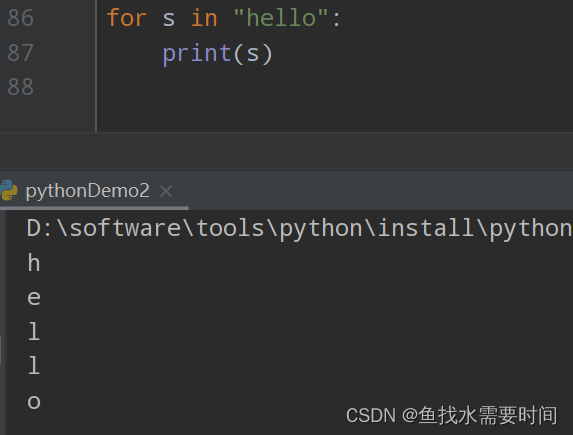### 7.4.2 range

range 可以生成数字供 for 循环遍历,它可以传递三个参数，分别表示 起始、结束和步长。

• 打印数字
• ``````for i in range(5):
print(i)
``````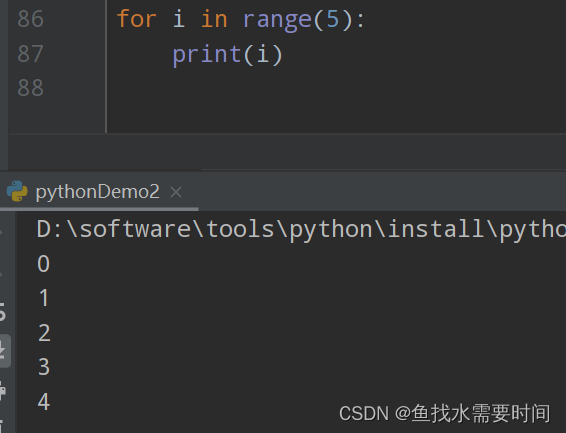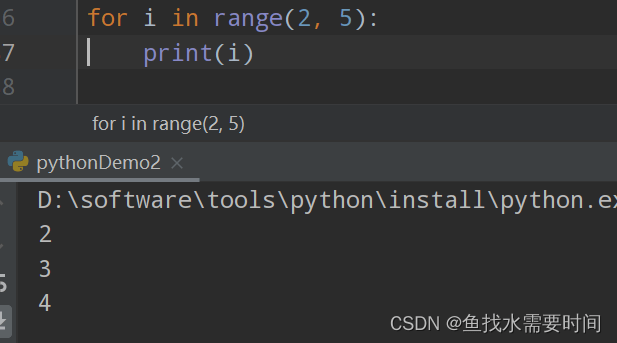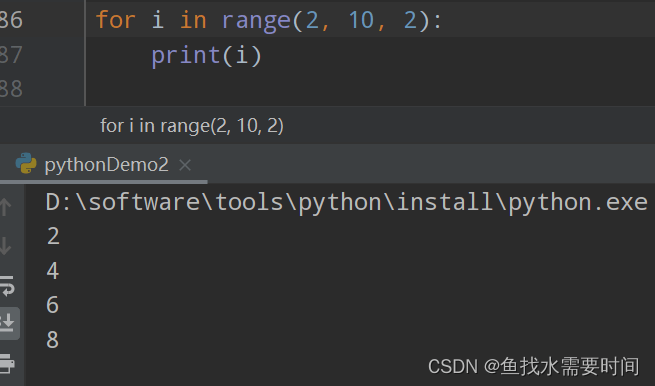打印九九乘法表

``````def f1(a):
for i in range(1, a+1):
s = ''
for j in range(1, i+1):
s += ('%d*%d=%d   ' % (j, i, i*j))
print(s)

f1(9);
``````

结果：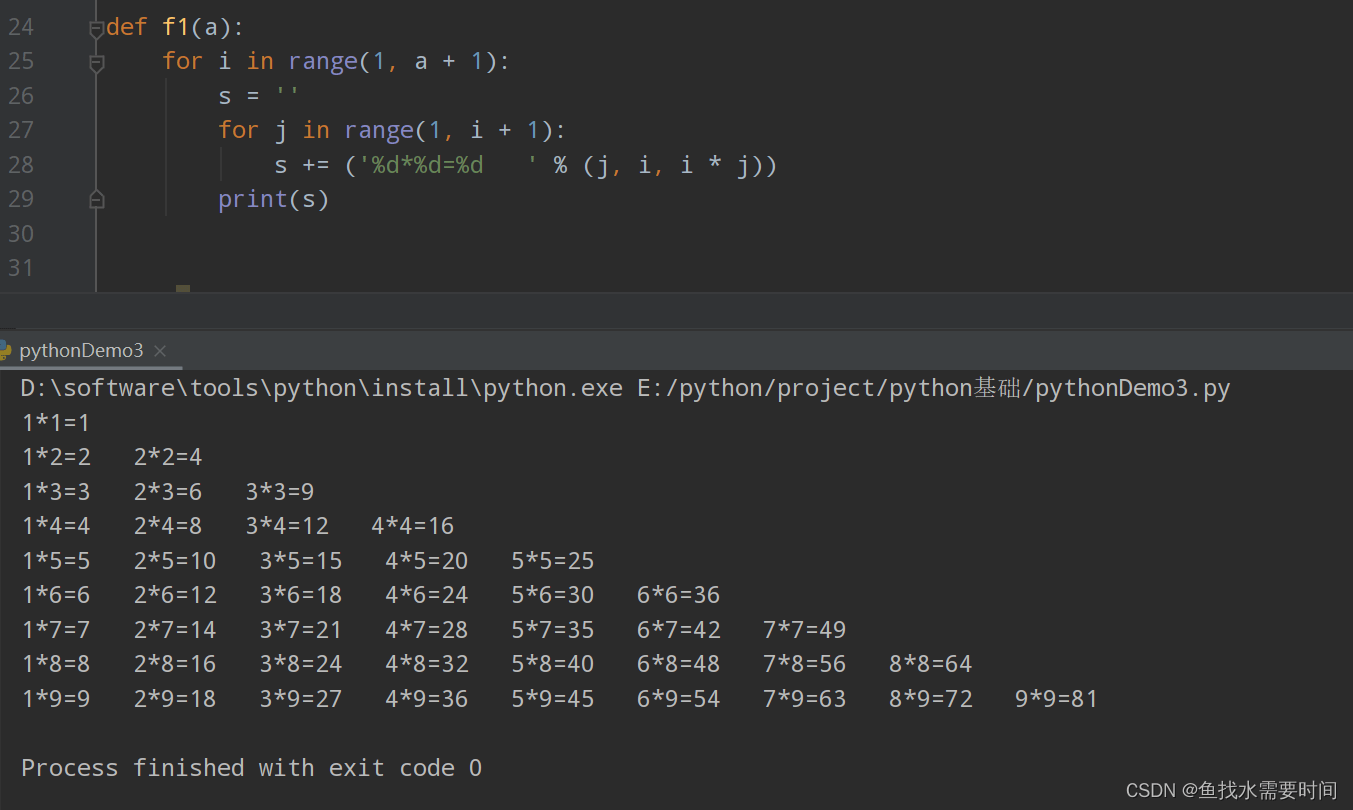打印菱形

``````def f1(a):
# 获取中位数,即取整除
z = a // 2
# 根据参数a循环
for i in range(a):
# 空格的数量：中位数-当前循环数
b = z - i
# 如果b<0,则空格数等于绝对值
if b < 0:
b = 0 - b
k = ' '
# 计算空格数量
k = b * k
c = '*'
# 参数a减去两边空格的数量就是*号的数量
c = (a - b * 2) * c
print(k + c + k)

f1(17)
``````

结果：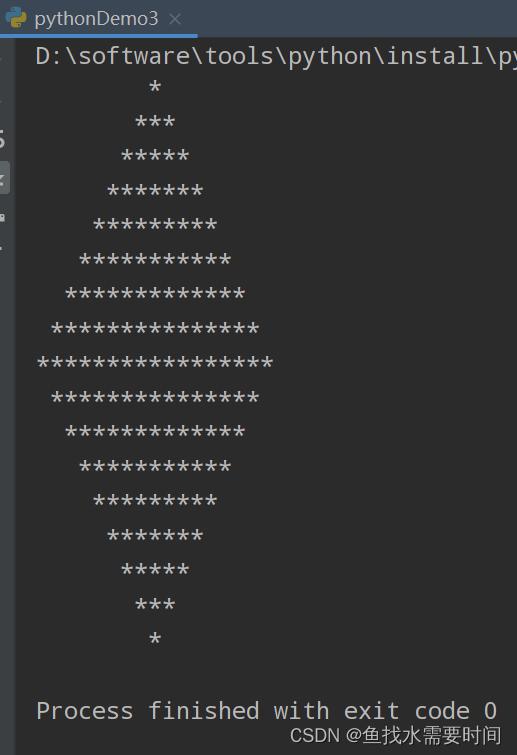### 7.4.3 循环列表

``````a_list = ['张三', '李四', '王五']
for i in a_list:
print(i)
``````

Python基础语法入门篇(二)

来源：鱼找水需要时间

物联沃分享整理
物联沃-IOTWORD物联网 » Python基础语法入门篇(一)